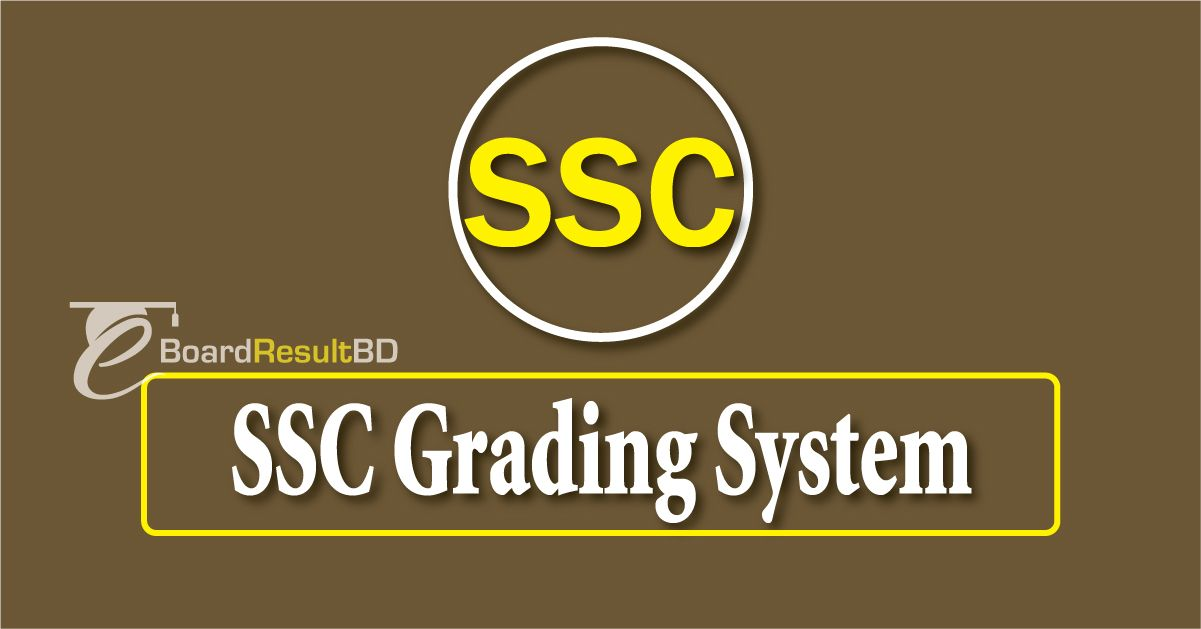SSC Grading System 2019, SSC Marking Method in Bangladesh. SSC result 2019 which will be declared on 5th May 2019 is provided based on the SSC GPA which is Grade Point Average that the students scored in the exam. If you want to know more about the SSC Grading System 2019, you must continue reading our Article. “GPA” which is also called as Grade Point Average is along with the procedure to calculate the GPA of the SSC result using this Grading system.

From past few years, the SSC Exam results are being announced based in the GPA system or grading system i.e., the results are given in the GPA format for the contenders. Many students and their guardians do not know what exactly grading system is and how to calculate GPA for SSC and to help them we have given the example below the grading system table given here. We advice the students to go through it to get an overview of it and to calculate your exam result of SSC and Dakhil examinations for the year 2019.

### Grading System for SSC Exams 2019

 Marks Range Grade Points Grade 80 – 100 5.00 A+ 70 – 79 4.00 A 60 – 69 3.50 A- 50 – 59 3.00 B 40 – 49 2.00 C 33 – 39 1.00 D 0 – 32 0.00 F

There will be a total of 7 grades from A+ to F and the grade points from 5 to 0 in this SSC Grading system of the education board. All the theoretical, practical and MCQ results are given in the Grading system in Bangladesh public exam results. For every subject, the student will be given a grade point and the grade for the marks obtained by the student in that particular subject. Finally, the average of the all grade points is taken and the overall grade point is assigned to the students and for which the grade is awarded for the candidates as a SSC Result 2019 for the public examinations conducted in that year.

## How to Calculate GPA for SSC?

Here, Is a Sample Grade Point Making System of a SSC Examine 2019, with his Subject Wise Grade/Point. In the Sample Copy, Just add your Expected Letter Grade beside the Subject code and Subject Name. Then follow the below Instruction to make your GPA.

 Subject Code Subject Name Grade Point 101 BANGLA A+ 5.00 107 ENGLISH A+ 5.00 109 MATHEMATICS A+ 5.00 145 SOCIAL SCIENCE A 4.00 111 ISLAM AND MORAL EDUCATION A 4.00 136 PHYSICS A+ 5.00 137 CHEMISTRY A 4.00 138 BIOLOGY A 4.00 131 COMPUTER STUDIES (Optional) A+ 5.00

Total Point: (5.00+5.00+5.00+4.00+4.00+5.00+4.00+4.00+5.00) = 41.00-2.00 (For Optional Subject) = 39.00

So, Grade Point Average (GPA) = 39.00/8= 4.875 or, 4.88

Here, 8= Total Subject Number. With the Above system, you can easily make Your Point Table of Education Board Bangladesh Public Exam Result.

For example: If the student scores 79 marks in particular subject, he/she will get the Grade point 4.00 and the grade as A. If the score is 80, the grade point is 5.00 and the grade is A+. Based on the score obtained one can check grade points from the table and calculate the overall grade and grade point of the overall score obtained by the student in the SSC Exams 2019 or Dakhil exams 2019.

GPA = Addition of Grade Points in Each Subject / Total Number of Subjects

This is how the SSC and DAKHIL result 2019 are given in the grading system for the students. The result will be published for the student in GPA with grades and grade points and the marks in each subject and the overall score will be available with the respective schools from which students can check their score.

### SSC Exams Marks Distribution

The education board ministry has changed the distribution of marks in the SSC Exams and students should be aware of this. NCBT has finalized the new syllabus as well as the new marks distribution system for the SSC Exam 2019 and its equivalent examinations.